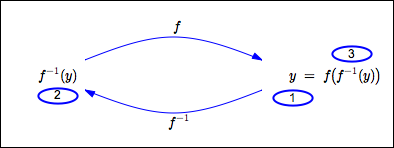﻿ Properties of Inverse Functions

#Properties of Inverse Functions

This lesson will be more accessible if you fully understand the concepts in these prior lessons:

Every one-to-one function $\,f\,$ has an inverse. This inverse is denoted by $\,f^{-1}\,$ and read aloud as ‘$\,f\,$ inverse’.

A function and its inverse ‘undo’ each other: one function does something, the other undoes it. The purpose of this lesson is to make this idea precise.

## Review: Domain and Range of a Function

The set of allowable inputs for a function $\,f\,$ is called its domain, and is denoted by $\,\text{dom}(f)\,.$

The set of all possible outputs from a function $\,f\,$ is called its range, and is denoted by $\,\text{ran}(f)\,.$ That is, let $\,f\,$ act on every possible input—every element of its domain. The set of resulting outputs is the range of $\,f\,$:

$$\cssId{s19}{\text{ran}(f)} \cssId{s20}{= \{ f(x)\ |\ x\in\text{dom}(f) \}}$$

## The Functions $\,f\,$ and $\,f^{-1}\,$ ‘Undo’ Each Other1. Start with an allowable input for $\,f\,.$ That is, let $\,x\in\text{dom}(f)\,.$
2. Let $\,f\,$ act on $\,x\,,$ giving $\,f(x)\,.$
3. Let $\,f^{-1}\,$ act on $\,f(x)\,,$ giving $\,f^{-1}(f(x))\,.$

This returns us to where we started, so:

$\,f^{-1}(f(x)) = x\,$ for all $\,x\,$ in the domain of $\,f\,$

In other words, the composite function $\,f^{-1}\circ f\,$ is the identity function on $\,\text{dom}(f)\,.$1. Start with an output from $\,f\,.$ That is, let $\,y\in\text{ran}(f)\,.$
2. Let $\,f^{-1}\,$ act on $\,y\,,$ giving $\,f^{-1}(y)\,.$
3. Let $\,f\,$ act on $\,f^{-1}(y)\,,$ giving $\,f\bigl(f^{-1}(y)\bigr)\,.$

This returns us to where we started, so:

$\,f\bigl(f^{-1}(y)\bigr) = y\,$ for all $\,y\,$ in the range of $\,f\,$

In other words, the composite function $\,f\circ f^{-1}\,$ is the identity function on $\,\text{ran}(f)\,.$

## Input/Output Roles Reversed for $\,f\,$ and $\,f^{-1}\,$

The input/output roles for a function and its inverse are reversedthe inputs to one are the outputs from the other. This fact has some nice consequences:

### Points on the Graphs of $\,f\,$ and $\,f^{-1}\,$ Have Their Coordinates Switched

$\,(a,b)\,$ is on the graph of $\,f\,$
if and only if
$\,(b,a)\,$ is on the graph of $\,f^{-1}\,$

### Domain and Range of $\,f^{-1}$

• The inputs to $\,f^{-1}\,$ are the outputs from $\,f\,.$ That is, $\,\text{dom}(f^{-1}) = \text{ran}(f)\,.$
• The outputs from $\,f^{-1}\,$ are the inputs to $\,f\,.$ That is, $\,\text{ran}(f^{-1}) = \text{dom}(f)\,.$

## Summary: Properties of Inverse Functions

For your convenience, the properties of inverse functions discussed in this and earlier exercises are summarized below.

PROPERTIES OF INVERSE FUNCTIONS

A function $\,g\,$ has an inverse if and only if $\,g\,$ is a one-to-one function.

Let $\,f\,$ be a one-to-one function. The inverse of $\,f\,$ is denoted by $\,f^{-1}\,$ and read aloud as ‘$\,f\,$ inverse’.

The functions $\,f\,$ and $\,f^{-1}\,$ satisfy the following properties:

• $\,f^{-1}\,$ is also a one-to-one function
• the inverse of $\,f^{-1}\,$ is $\,f\,$
• $\,f(x) = y\,$  if and only if  $\,f^{-1}(y) = x$
• $\,f^{-1}(f(x)) = x\,$  for all  $\,x\in\text{dom}(f)$
• $\,f\bigl(f^{-1}(y)\bigr) = y\,$  for all  $\,y\in\text{ran}(f)$
• $(a,b)$ is on the graph of $\,f\,$  if and only if  $\,(b,a)\,$ is on the graph of $\,f^{-1}$
• $\text{dom}(f^{-1}) = \text{ran}(f)\,$  and  $\text{ran}(f^{-1}) = \text{dom}(f)$